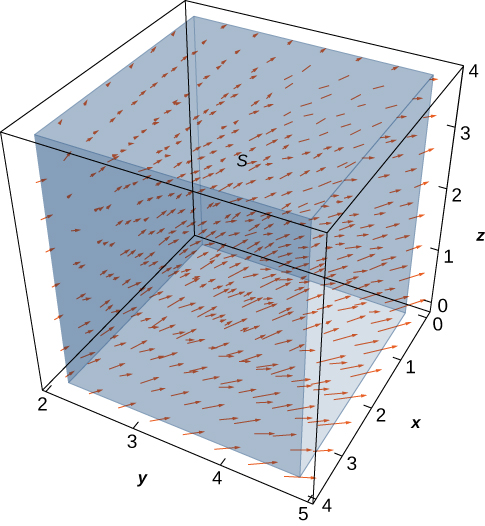# 6.8 The divergence theorem  (Page 4/12)

 Page 4 / 12

Let $\text{v}=⟨\frac{x}{z},\frac{y}{z},0⟩$ be the velocity field of a fluid. Let C be the solid cube given by $1\le x\le 4,2\le y\le 5,1\le z\le 4,$ and let S be the boundary of this cube (see the following figure). Find the flow rate of the fluid across S .$9\phantom{\rule{0.2em}{0ex}}\text{ln}\left(16\right)$

[link] illustrates a remarkable consequence of the divergence theorem. Let S be a piecewise, smooth closed surface and let F be a vector field defined on an open region containing the surface enclosed by S . If F has the form $\text{F}=⟨f\left(y,z\right),g\left(x,z\right),h\left(x,y\right)⟩,$ then the divergence of F is zero. By the divergence theorem, the flux of F across S is also zero. This makes certain flux integrals incredibly easy to calculate. For example, suppose we wanted to calculate the flux integral ${\iint }_{S}\text{F}·d\text{S}$ where S is a cube and

$\text{F}=⟨\text{sin}\left(y\right){e}^{yz},{x}^{2}{z}^{2},\text{cos}\left(xy\right){e}^{\text{sin}\phantom{\rule{0.2em}{0ex}}x}⟩.$

Calculating the flux integral directly would be difficult, if not impossible, using techniques we studied previously. At the very least, we would have to break the flux integral into six integrals, one for each face of the cube. But, because the divergence of this field is zero, the divergence theorem immediately shows that the flux integral is zero.

We can now use the divergence theorem to justify the physical interpretation of divergence that we discussed earlier. Recall that if F is a continuous three-dimensional vector field and P is a point in the domain of F , then the divergence of F at P is a measure of the “outflowing-ness” of F at P . If F represents the velocity field of a fluid, then the divergence of F at P is a measure of the net flow rate out of point P (the flow of fluid out of P less the flow of fluid in to P ). To see how the divergence theorem justifies this interpretation, let ${B}_{r}$ be a ball of very small radius r with center P , and assume that ${B}_{r}$ is in the domain of F . Furthermore, assume that ${B}_{r}$ has a positive, outward orientation. Since the radius of ${B}_{r}$ is small and F is continuous, the divergence of F is approximately constant on ${B}_{r}.$ That is, if ${P}^{\prime }$ is any point in ${B}_{r},$ then $\text{div}\phantom{\rule{0.2em}{0ex}}\text{F}\left(P\right)\approx \text{div}\phantom{\rule{0.2em}{0ex}}\text{F}\left({P}^{\prime }\right).$ Let ${S}_{r}$ denote the boundary sphere of ${B}_{r}.$ We can approximate the flux across ${S}_{r}$ using the divergence theorem as follows:

$\begin{array}{cc}\hfill {\iint }_{{S}_{r}}\text{F}·d\text{S}& ={\iiint }_{{B}_{r}}\text{div}\phantom{\rule{0.2em}{0ex}}\text{F}\phantom{\rule{0.2em}{0ex}}dV\hfill \\ & \approx {\iiint }_{{B}_{r}}\text{div}\phantom{\rule{0.2em}{0ex}}\text{F}\left(P\right)dV\hfill \\ & =\text{div}\phantom{\rule{0.2em}{0ex}}\text{F}\left(P\right)V\left({B}_{r}\right).\hfill \end{array}$

As we shrink the radius r to zero via a limit, the quantity $\text{div}\phantom{\rule{0.2em}{0ex}}\text{F}\left(P\right)V\left({B}_{r}\right)$ gets arbitrarily close to the flux. Therefore,

$\text{div}\phantom{\rule{0.2em}{0ex}}\text{F}\left(P\right)=\underset{r\to 0}{\text{lim}}\frac{1}{V\left({B}_{r}\right)}{\iint }_{{S}_{r}}\text{F}·d\text{S}$

and we can consider the divergence at P as measuring the net rate of outward flux per unit volume at P . Since “outflowing-ness” is an informal term for the net rate of outward flux per unit volume, we have justified the physical interpretation of divergence we discussed earlier, and we have used the divergence theorem to give this justification.

## Application to electrostatic fields

The divergence theorem has many applications in physics and engineering. It allows us to write many physical laws in both an integral form and a differential form (in much the same way that Stokes’ theorem allowed us to translate between an integral and differential form of Faraday’s law). Areas of study such as fluid dynamics, electromagnetism, and quantum mechanics have equations that describe the conservation of mass, momentum, or energy, and the divergence theorem allows us to give these equations in both integral and differential forms.

general equation for photosynthesis
6CO2 + 6H2O + solar energy= C6H1206+ 6O2
Anastasiya
meaning of amino Acids
a diagram of an adult mosquito
what are white blood cells
white blood cell is part of the immune system. that help fight the infection.
MG
Mlungisi
Cells with a similar function, form a tissue. For example the nervous tissue is composed by cells:neurons and glia cells. Muscle tissue, is composed by different cells.
Anastasiya
I need further explanation coz celewi anything guys,,,
hey guys
Isala
on what?
Anastasiya
hie
Lish
is air homogenous or hetrogenous
homogenous
Kevin
why saying homogenous?
Isala
explain if oxygen is necessary for photosynthesis
explain if oxygen is necessary for photosynthesis
Yes, the plant does need oxygen. The plant uses oxygen, water, light, and produced food. The plant use process called photosynthesis.
MG
By using the energy of sunlight, plants convert carbon dioxide and water into carbohydrates and oxygen by photosynthesis. This happens during the day and sunlight is needed.
NOBLE
no. it s a product of the process
Anastasiya
yet still is it needed?
NOBLE
no. The reaction is: 6CO2+6H20+ solar energy =C6H12O6(glucose)+602. The plant requires Carbon dioxyde, light, and water Only, and produces glucose and oxygen( which is a waste).
Anastasiya
what was the question
joining
Godfrey
the specific one
NOBLE
the study of non and living organism is called.
Godfrey
Is call biology
Alohan
yeah
NOBLE
yes
Usher
what Is ecology
what is a cell
A cell is a basic structure and functional unit of life
Ndongya
what is biolgy
is the study of living and non living organisms
Ahmed
may u draw the female organ
i dont understand
Asal
:/
Asal
me too
DAVID
anabolism and catabolism
Anabolism refers to the process in methabolism in which complex molecules are formed "built" and requires energy to happen. Catabolism is the opposite process: complex molecules are deconstructed releasing energy, such as during glicolysis.
Anastasiya
Explain briefly independent assortment gene .
hi
Amargo
hi I'm Anatalia
Joy
what do you mean by pituitary gland
Digambar
Got questions? Join the online conversation and get instant answers!By OpenStaxBy Madison ChristianBy Stephen VoronBy OpenStaxBy OpenStaxByBy OpenStaxBy Michael SagBy Marion CabalfinBy Lakeima Roberts